## Audio

[Audio][slideshow]

## Basic Flip Flops

This article deals with the basic flip flop circuits like S-R Flip Flop, J-K Flip Flop, D Flip Flop, and T Flip Flop along with truth tables and their corresponding circuit symbols.

Before going to the topic it is important that you get knowledge of its basics. Click on the links below for more information.

TAKE A LOOK : BOOLEAN LOGIC

TAKE A LOOK : LOGIC GATES

Flip flops are actually an application of logic gates. With the help of Boolean logic you can create memory with them. Flip flops can also be considered as the most basic idea of a Random Access Memory [RAM]. When a certain input value is given to them, they will be remembered and executed, if the logic gates are designed correctly. A higher application of flip flops is helpful in designing better electronic circuits.

The most commonly used application of flip flops is in the implementation of a feedback circuit. As a memory relies on the feedback concept, flip flops can be used to design it.

There are mainly four types of flip flops that are used in electronic circuits. They are

1. The basic Flip Flop or S-R Flip Flop
2. Delay Flip Flop [D Flip Flop]
3. J-K Flip Flop
4. T Flip Flop

## 1. S-R Flip Flop

The SET-RESET flip flop is designed with the help of two NOR gates and also two NAND gates. These flip flops are also called S-R Latch.

• ### S-R Flip Flop using NOR Gate

The design of such a flip flop includes two inputs, called the SET [S] and RESET [R]. There are also two outputs, Q and Q’. The diagram and truth table is shown below.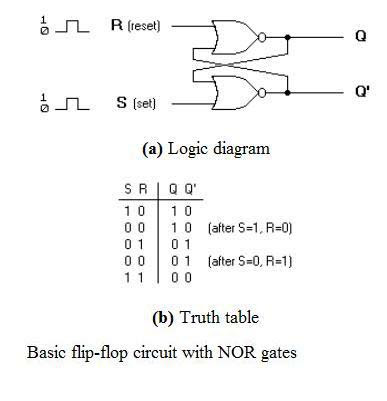S-R Flip Flop using NOR Gate

From the diagram it is evident that the flip flop has mainly four states. They are

S=1, R=0—Q=1, Q’=0

This state is also called the SET state.

S=0, R=1—Q=0, Q’=1

This state is known as the RESET state.

In both the states you can see that the outputs are just compliments of each other and that the value of Q follows the value of S.

S=0, R=0—Q & Q’ = Remember

If both the values of S and R are switched to 0, then the circuit remembers the value of S and R in their previous state.

S=1, R=1—Q=0, Q’=0 [Invalid]

This is an invalid state because the values of both Q and Q’ are 0. They are supposed to be compliments of each other. Normally, this state must be avoided.

• ### S-R Flip Flop using NAND Gate

The circuit of the S-R flip flop using NAND Gate and its truth table is shown below.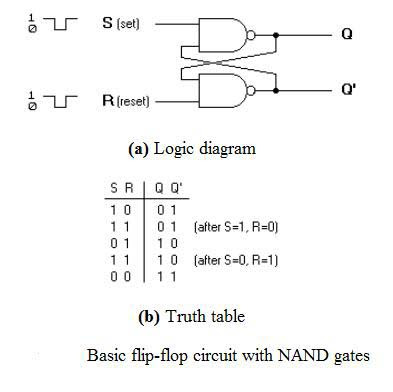S-R Flip Flop using NAND Gate

Like the NOR Gate S-R flip flop, this one also has four states. They are

S=1, R=0—Q=0, Q’=1

This state is also called the SET state.

S=0, R=1—Q=1, Q’=0

This state is known as the RESET state.

In both the states you can see that the outputs are just compliments of each other and that the value of Q follows the compliment value of S.

S=0, R=0—Q=1, & Q’ =1 [Invalid]

If both the values of S and R are switched to 0 it is an invalid state because the values of both Q and Q’ are 1. They are supposed to be compliments of each other. Normally, this state must be avoided.

S=1, R=1—Q & Q’= Remember

If both the values of S and R are switched to 1, then the circuit remembers the value of S and R in their previous state.

• ### Clocked S-R Flip Flop

It is also called a Gated S-R flip flop.

The problems with S-R flip flops using NOR and NAND gate is the invalid state. This problem can be overcome by using a bistable SR flip-flop that can change outputs when certain invalid states are met, regardless of the condition of either the Set or the Reset inputs. For this, a clocked S-R flip flop is designed by adding two AND gates to a basic NOR Gate flip flop. The circuit diagram and truth table is shown below.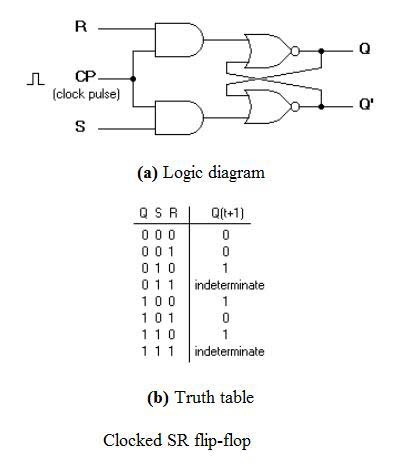Clocked S-R Flip Flop

A clock pulse [CP] is given to the inputs of the AND Gate. When the value of the clock pulse is ‘0’, the outputs of both the AND Gates remain ‘0’. As soon as a pulse is given the value of CP turns ‘1’. This makes the values at S and R to pass through the NOR Gate flip flop. But when the values of both S and R values turn ‘1’, the HIGH value of CP causes both of them to turn to ‘0’ for a short moment. As soon as the pulse is removed, the flip flop state becomes intermediate. Thus either of the two states may be caused, and it depends on whether the set or reset input of the flip-flop remains a ‘1’ longer than the transition to ‘0’ at the end of the pulse. Thus the invalid states can be eliminated.

## 2. D Flip Flop

The circuit diagram and truth table is given below.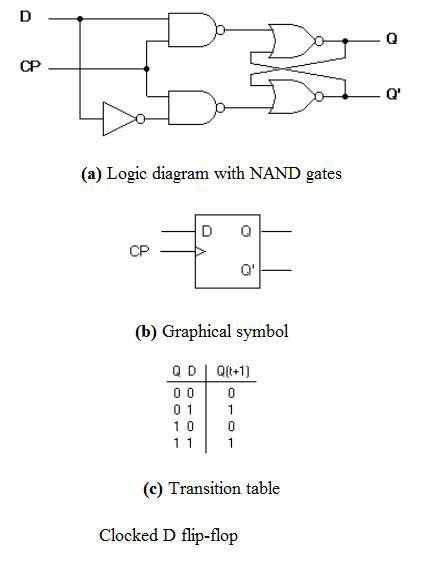D Flip Flop

D flip flop is actually a slight modification of the above explained clocked SR flip-flop. From the figure you can see that the D input is connected to the S input and the complement of the D input is connected to the R input. The D input is passed on to the flip flop when the value of CP is ‘1’. When CP is HIGH, the flip flop moves to the SET state. If it is ‘0’, the flip flop switches to the CLEAR state.

To know more about the triggering of flip flop click on the link below.

TAKE A LOOK : TRIGGERING OF FLIP FLOPS

TAKE A LOOK : MASTER-SLAVE FLIP FLOP CIRCUIT

## 3. J-K Flip Flop

The circuit diagram and truth-table of a J-K flip flop is shown below.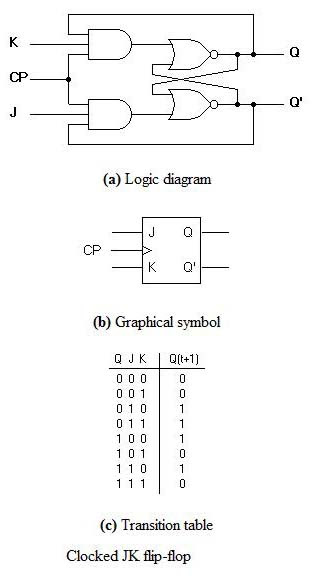J-K Flip Flop

A J-K flip flop can also be defined as a modification of the S-R flip flop. The only difference is that the intermediate state is more refined and precise than that of a S-R flip flop.

The behavior of inputs J and K is same as the S and R inputs of the S-R flip flop. The letter J stands for SET and the letter K stands for CLEAR.

When both the inputs J and K have a HIGH state, the flip-flop switch to the complement state. So, for a value of Q = 1, it switches to Q=0 and for a value of Q = 0, it switches to Q=1.

The circuit includes two 3-input AND gates. The output Q of the flip flop is returned back as a feedback to the input of the AND along with other inputs like K and clock pulse [CP]. So, if the value of CP is ‘1’, the flip flop gets a CLEAR signal and with the condition that the value of Q was earlier 1. Similarly output Q’ of the flip flop is given as a feedback to the input of the AND along with other inputs like J and clock pulse [CP]. So the output becomes SET when the value of CP is 1 only if the value of Q’ was earlier 1.

The output may be repeated in transitions once they have been complimented for J=K=1 because of the feedback connection in the JK flip-flop. This can be avoided by setting a time duration lesser than the propagation delay through the flip-flop. The restriction on the pulse width can be eliminated with a master-slave or edge-triggered construction.

## 4. T Flip Flop

This is a much simpler version of the J-K flip flop. Both the J and K inputs are connected together and thus are also called a single input J-K flip flop. When clock pulse is given to the flip flop, the output begins to toggle. Here also the restriction on the pulse width can be eliminated with a master-slave or edge-triggered construction. Take a look at the circuit and truth table below.T Flip Flop

[Lights][grids]